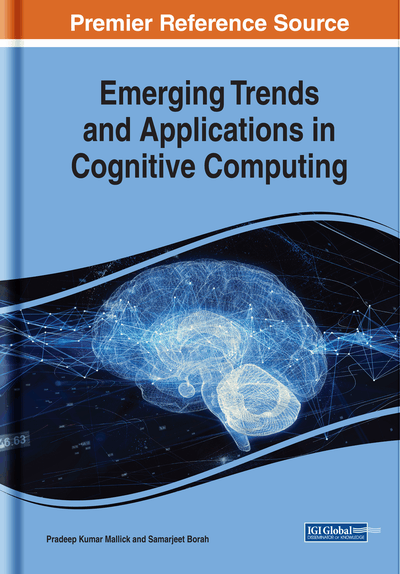# Neuro-Fuzzy Models and Applications

Sushruta Mishra (KIIT University, India), Soumya Sahoo (C. V. Raman College of Engineering, India) and Brojo Kishore Mishra (C. V. Raman College of Engineering, India)
DOI: 10.4018/978-1-5225-5793-7.ch004

## Abstract

The modern techniques of artificial intelligence have found application in almost all the fields of human knowledge. Among them, two important techniques of artificial intelligence, fuzzy systems (FS) and artificial neural networks (ANNs), have found many applications in various fields such as production, control systems, diagnostic, supervision, etc. They evolved and improved throughout the years to adapt arising needs and technological advancements. However, a great emphasis is given in the engineering field. The techniques of artificial intelligence based on fuzzy logic and neural networks are frequently applied together for solving engineering problems where the classic techniques do not supply an easy and accurate solution. Separately, each one of these techniques possesses advantages and disadvantages that, when mixed together, provide better results than the ones achieved with the use of each isolated technique. As ANNs and fuzzy systems have often been applied together, the concept of a fusion between them started to take shape. Neuro-fuzzy systems were born which utilize the advantages of both techniques. Such systems show two distinct ways of behavior. In a first phase, called learning phase, it behaves like neural networks that learn internal parameters off-line. Later, in the execution phase, it behaves like a fuzzy logic system. A neuro-fuzzy system is a fuzzy system that uses a learning algorithm derived from or inspired by neural network theory to determine its parameters (fuzzy sets and fuzzy rules) by processing data samples. Neural networks and fuzzy systems can be combined to join its advantages and to cure its individual illness. Neural networks introduce its computational characteristics of learning in the fuzzy systems and receive from them the interpretation and clarity of systems representation. Thus, the disadvantages of the fuzzy systems are compensated by the capacities of the neural networks. These techniques are complementary, which justifies its use together. This chapter deals with an analysis of neuro-fuzzy systems. Benefits of these systems are studied with its limitations too. Comparative analyses of various categories of neuro-fuzzy systems are discussed in detail. Apart from these, real-time applications of such systems are also presented.
Chapter Preview
Top

## Background

Fuzzy systems propose a mathematic calculus to translate the subjective human knowledge of the real processes. This is a way to manipulate practical knowledge with some level of uncertainty. The fuzzy sets theory was initiated by the authors (Tano et al., 1996) The behavior of such systems is described through a set of fuzzy rules, like:IF <Premise> THEN <Consequent> that uses linguistics variables with symbolic terms. Each term represents a fuzzy set. The terms of the input space (typically 5-7 for each linguistic variable) compose the fuzzy partition. The fuzzy inference mechanism consists of three stages: in the first stage, the values of the numerical inputs are mapped by a function according to a degree of compatibility of the respective fuzzy sets; this operation can be called fuzzification. In the second stage, the fuzzy system processes the rules in accordance with the firing strengths of the inputs. In the third stage, the resultant fuzzy values are transformed again into numerical values; this operation can be called defuzzyfication. Essentially, this procedure makes possible the use fuzzy categories in representation of words and abstracts ideas of the human beings in the description of the decision taking procedure.

• Capacity to represent inherent uncertainties of the human knowledge with linguistic variables.

• Simple interaction of the expert of the domain with the engineer designer of the system.

• Easy interpretation of the results, because of the natural rules representation.

• Easy extension of the base of knowledge through the addition of new rules.

• Robustness in relation of the possible disturbances in the system.